# Transmission rate of a channel

(diff) ← Older revision | Latest revision (diff) | Newer revision → (diff)

An information-theoretic measure of the ability to transmit information over a communication channel. Letandbe random variables connected in a communication channel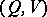. Then the transmission rateof this channel is defined by the equation(1)

where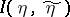is the amount of information (cf. Information, amount of) in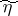relative to, and the supremum is taken over all pairs of random variablesconnected in the channel. In the case when the input and output signals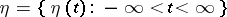and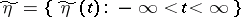are random processes in continuous or discrete time, the transmission rate of the channel is usually understood to mean the mean transmission rate of the channel, taken in unit time or over one symbol of the transmitted signal, that is, by definition one sets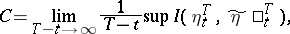(2)

if the limit exists; here the supremum is taken over all possible pairs of random variables,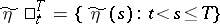connected in the corresponding segment of the given channel. The existence of the limit (2) has been proved for a wide class of channels, for example for a homogeneous channel with a finite memory and non-vanishing transition probabilities.

It is known that for a sufficiently wide class (for example, for the channels with finite memory mentioned above) the following holds: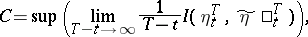(3)

where the supremum is taken over all pairs of stationarily-related random processes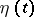,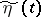,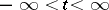, such that for any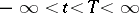the random variablesandare connected in the corresponding segment of the channel under consideration. Thus, (3) shows that the transmission rate of the channel is the same as the maximum possible transmission rate of information (cf. Information, transmission rate of) along this channel.

An explicit calculation of transmission rates is therefore of considerable interest. For example, for a channelwhose input and output signals take values in the Euclidean-dimensional space, with transition function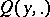defined by a density(with respect to the Lebesgue measure),, and with the constraintconsisting of boundedness of the mean square power of the input signal,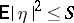(where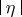is the length of the vectorin),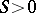being a fixed constant, the following results are known (see ).

1) Let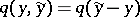, that is, one considers a channel with additive noise such that the output signalis equal to the sumof the input signaland a noise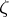independent of it, and let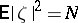. Then as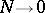(under weak additional conditions) the following asymptotic formula holds: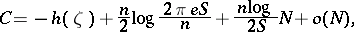whereis the differential entropy ofand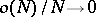as. This formula corresponds to the case of little noise.

2) Let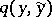be arbitrary but let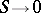. Then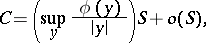where